Two transparent slabs have the same thickness as shown. One is made of material A of refractive index 1.5. The other is made of two materials B and C with thickness in the ratio 1:2. The refractive index of C is 1.6. If a monochromatic parallel beam passing through the slabs has the same number of waves inside both, the refractive index of B is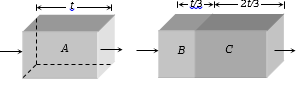(a)   1.1                                      (b)   1.2

(c)   1.3                                      (d)   1.4

Concept Questions :-

Refraction at plane surface
High Yielding Test Series + Question Bank - NEET 2020

Difficulty Level:

The image of point P when viewed from the top of the slabs will be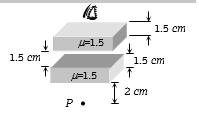(a)   2.0 cm above P

(b)   1.5 cm above P

(c)   2.0 cm below P

(d)   1 cm above P

Concept Questions :-

Refraction at plane surface
High Yielding Test Series + Question Bank - NEET 2020

Difficulty Level:

Two point light sources are 24 cm apart. Where should a convex lens of focal length 9 cm be put in between them from one source so that the images of both the sources are formed at the same place?

(a)   6 cm                                   (b)   9 cm

(c)   12 cm                                (d)   15 cm

Concept Questions :-

Lenses
High Yielding Test Series + Question Bank - NEET 2020

Difficulty Level:

(a)   2R                                      (b)   R

(c)   $\frac{3}{2}R$                                 (d)  ${R}^{2}$

Concept Questions :-

Lensmakers' formula
High Yielding Test Series + Question Bank - NEET 2020

Difficulty Level:

A prism having an apex angle 4o and refraction index 1.5 is located in front of a vertical plane mirror as shown in the figure. Through what total angle is the ray deviated after reflection from the mirror?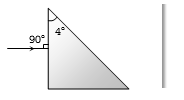(a)   176o

(b)   4o

(c)   178o

(d)   2o

Concept Questions :-

Prisms
High Yielding Test Series + Question Bank - NEET 2020

Difficulty Level:

A rectangular glass slab ABCD, of refractive index ${n}_{1}$, is immersed in water of the refractive index ${n}_{2}\left({n}_{1}>{n}_{2}\right)$. A ray of light is incident at the surface AB of the slab as shown. The maximum value of the angle of incidence ${\alpha }_{max}$, such that the ray comes out only from the other surface CD is given by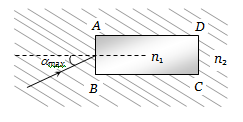(a) ${\mathrm{sin}}^{-1}\left[\frac{{n}_{1}}{{n}_{2}}\mathrm{cos}\left({\mathrm{sin}}^{-1}\frac{{n}_{2}}{{n}_{1}}\right)\right]$                 (b) ${\mathrm{sin}}^{-1}\left[{n}_{1}\mathrm{cos}\left({\mathrm{sin}}^{-1}\frac{1}{{n}_{2}}\right)\right]$

(c) ${\mathrm{sin}}^{-1}\left(\frac{{n}_{1}}{{n}_{2}}\right)$                                      (d) ${\mathrm{sin}}^{-1}\left(\frac{{n}_{2}}{{n}_{1}}\right)$

Concept Questions :-

Total internal reflection
High Yielding Test Series + Question Bank - NEET 2020

Difficulty Level:

A rod of glass ($\upsilon$ = 1.5) and of square cross section is bent into the shape shown in the figure. A parallel beam of light falls on the plane flat surface A as shown in the figure. If d is the width of a side and R is the radius of circular arc then for what maximum value of $\frac{d}{R}$  light entering the glass slab through surface A emerges from the glass through B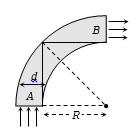(a)   1.5

(b)   0.5

(c)   1.3

(d)   None of these

Concept Questions :-

Total internal reflection
High Yielding Test Series + Question Bank - NEET 2020

Difficulty Level:

A diverging beam of light from a point source S having divergence angle $\alpha$, falls symmetrically on a glass slab as shown. The angles of incidence of the two extreme rays are equal. If the thickness of the glass slab is t and the refractive index n, then the divergence angle of the emergent beam is :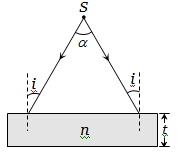(a) Zero                                       (b) $\alpha$

(c) ${\mathrm{sin}}^{-1}\left(1/n\right)$                           (d) $2{\mathrm{sin}}^{-1}\left(1/n\right)$

Concept Questions :-

Refraction at plane surface
High Yielding Test Series + Question Bank - NEET 2020

Difficulty Level:

A concave mirror is placed at the bottom of an empty tank with face upwards and axis vertical. When sunlight falls normally on the mirror, it is focused at distance of 32 cm from the mirror. If the tank is filled with water $\left(\mu =\frac{4}{3}\right)$ upto a height of 20 cm, then the sunlight will now get focussed at

(a) 16 cm above water level

(b) 9 cm above water level

(c) 24 cm below water level

(d) 9 cm below water level

Concept Questions :-

Refraction at plane surface
High Yielding Test Series + Question Bank - NEET 2020

Difficulty Level:

The slab of a material of refractive index 2 shown in figure has curved surface APB of radius of curvature 10 cm and a plane surface CD. On the left of APB is air and on the right of CD is water with refractive indices as given in figure. An object O is placed at a distance of 15 cm from pole P as shown. The distance of the final image of O from P, as viewed from the left is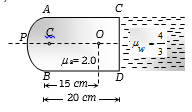(a)   20 cm

(b)   30 cm

(c)   40 cm

(d)   50 cm

Concept Questions :-

Lenses